# Kinetic theory of Bose Einstein Condensates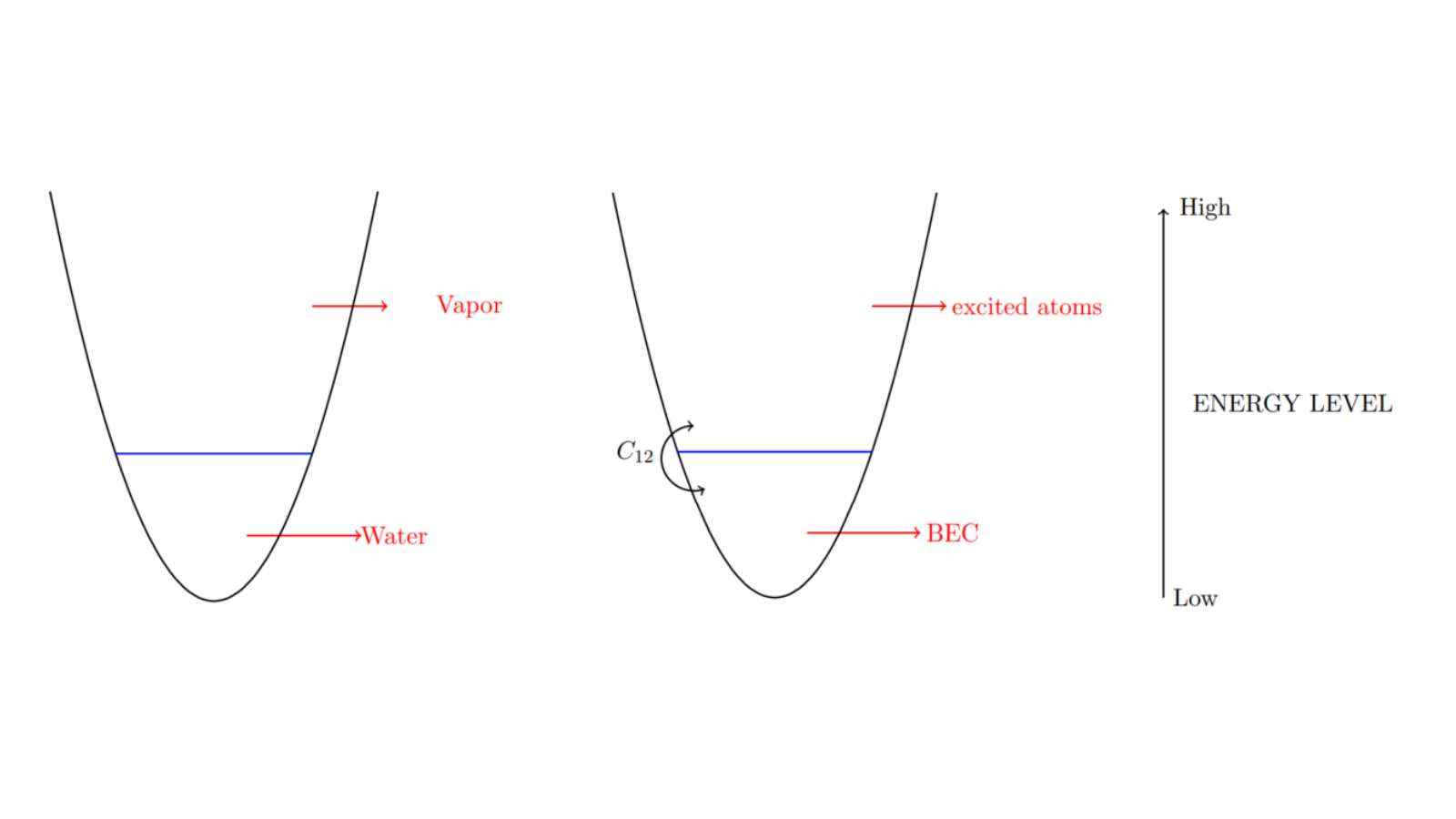## Kinetic theory of Bose Einstein Condensates

If a dilute gas of bosons, about one-hundred-thousandth the density of normal air, is cooled to a temperature very close to absolute zero (0 K or -273.15C), the gas will be changed into a new state of matter, called Bose-Einstein condensate (BEC). This state of matter was predicted by Satyendra Nath Bose and Albert Einstein in 1924-25.

In the pioneering MIT-BEC experiment (1998), which later led to the 2001 Nobel Prize in physics, one can observe the growth of the condensate after fast evaporative cooling, which cools the gas below the BEC transition temperature. The condensate growth is an interesting dynamical process.

“The growth of a condensate is an interesting dynamical process atoms must find the lowest energy state of the system, and long-range coherence has to be established. Experimentally, this process is observed after fast evaporative cooling, which cools the gas below the transition temperature for Bose Einstein condensation, but is faster than the growth of the condensate to its equilibrium size. A full theoretical description must include the condensate and its elementary excitations, and the interactions with the cloud of thermal atoms (those not part of the condensate). This quantum kinetics problem has been approached from the perspective of quantum optics, which models the condensation process after lasing; while condensed matter theorists have investigated the same process in terms of symmetry breaking and phase relaxation.” (James R. Anglin & Wolfgang Ketterle, Nature, 2002).

In order to understand the dynamics of BECs and their excited atoms by the kinetic theory point of view, let first us look at the an example of a glass of water (Figure 1).

The glass is divided into two parts: water and vapor. The energy of the whole system waver-vapor is increasing from low (water) to high (vapor). (Notice that besides the kinetic theory point of view, there are other ways of understanding the The same thing happens for a BEC (Figure 2).

We can see that the BEC occupies the lowest quantum state, at that point macroscopic quantum phenomena become apparent. Above the BEC are excited atoms, which occupy higher quantum states. The are two types of interactions in this Figure 1:
• excited atoms – excited atoms,
• condensate atoms – excited atoms.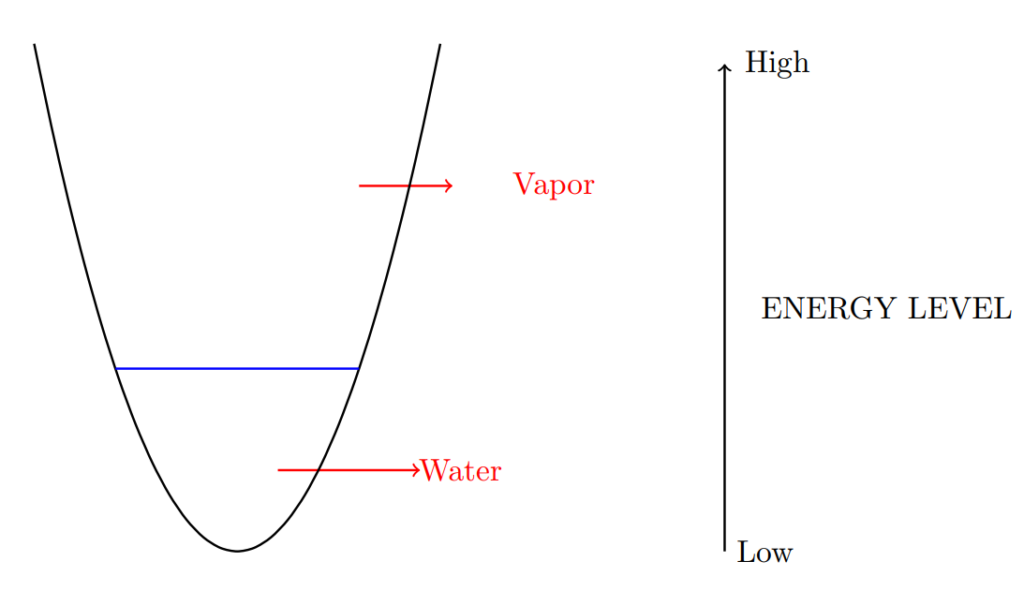Figure 1. The glass of water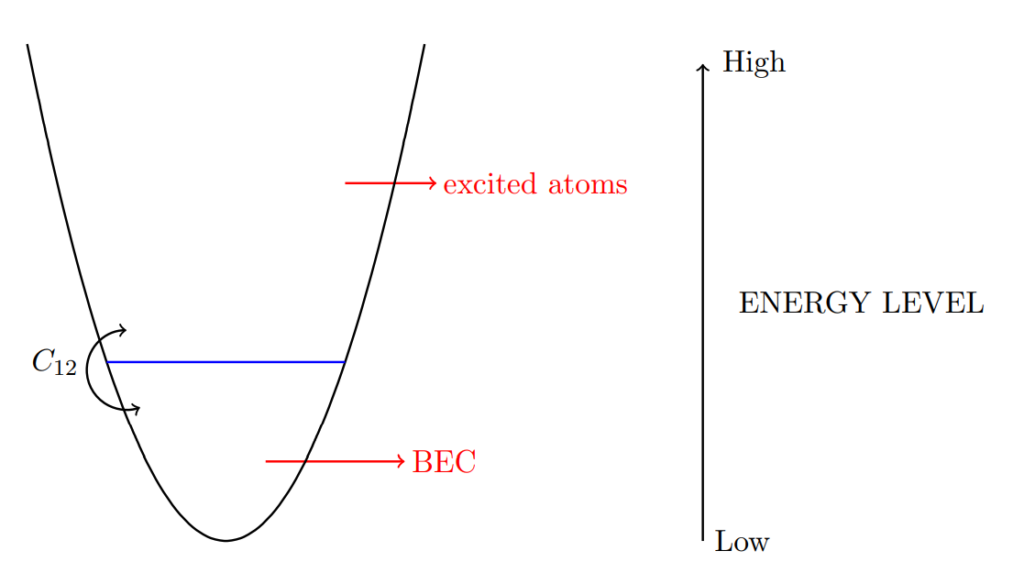Figure 2. The Bose-Einstein Condensate (BEC) and the excited atoms

“The behaviour of condensates at finite temperatures is a frontier of manybody physics, the experimental exploration of which has so far concentrated mainly on the initial formation of condensates.” (James R. Anglin & Wolfgang Ketterle, Nature, 2002)

Now, let us assume the following assumption, which facilitates the study of the BEC dynamics: Suppose the BEC is very stable and contains a sizable number of atoms, the interaction between excited atoms is small. As a consequence, the dominant interaction is the one between excited atoms and the BEC. We can see this in Figure 2. C_12 is the operator that describes the interaction between excited atoms and the condensate atoms.

The dynamics of the BEC in this case is governed by the following equation, where f(t,p) is the density distribution of excited atoms, at time t with momentum p:
where the interaction operator is defined as

\begin{aligned} C_{12}[f] := & \int_{\R^3} \int_{\R^3} \text{d}p_1 \text{d}p_2 \big[R(p, p_1, p_2) - R(p_1, p, p_2) - R(p_2, p_1, p) \big] , \\ & R(p, p_1, p_2):= \\ & \mathcal{M}(p, p_1, p_2)|^2\big[\delta (\mathcal{E}_p-\mathcal{E}_{p_1}-\mathcal{E}_{p_2}) \delta (p-p_1-p_2) \big] \times \big[ f(p_1)f(p_2)(1+f(p))-(1+f(p_1)(1+f(p_2))f(p) \big]. \end{aligned} (2)

Let us try to explain why the condensate growth term C_{12} has the form of (1). In order to understand this, we start with the collision operator for classical particles. Suppose that we need to study the density of a spacially homogeneous gas, whose distribution function is f(t,p), which indicates the probability of finding a particle with momentum p and time t. Now, suppose the two particles with momenta p_1 and p_2 collide and they change their momenta into p_3 and p_4. That means that we lose two particles with momenta p_1 and p_2, and gain two new particles with momenta p_3 and p_4 (see Figure 3). The probability of losing two particles with momenta p_1 and p_2 is f(t,p_1)f(t,p_2) and of gaining two particles with momenta p_3 and p_4 is f(t,p_3)f(t,p_3). As a result, the collision operator has to contain f(t,p_3)f(t,p_4)-f(t,p_1)f(t,p_2). Since the collision conserves mass and momentum, we also have p_1+p_2=p_3+p_4 and \mathcal{E}_{p_1} + \mathcal{E}_{p_2}= \mathcal{E}_{p_3} + \mathcal{E}_{p_4}, in which \mathcal{E}_p is the energy of the particle with momentum p. Finally the collision operator has to contain \delta(p_1+p_2-p_3-p_4)\delta(\mathcal{E}_{p_1} + \mathcal{E}_{p_2}- \mathcal{E}_{p_3} - \mathcal{E}_{p_4})[f(t,p_3)f(t,p_4)-f(t,p_1)f(t,p_2)]. The classical Boltzmann equation then reads
\begin{aligned} &\ \frac{\text{d}f}{\text{d}t}(t,p_1) \\ = &\ \int_{\mathbb{R}^9}K(p_1,p_2,p_3,p_4)\delta(p_1+p_2-p_3-p_4)\delta(\mathcal{E}_{p_1} + \mathcal{E}_{p_2}- \mathcal{E}_{p_3} - \mathcal{E}_{p_4})\times \\ & \ \times[f(t,p_3)f(t,p_4)-f(t,p_1)f(t,p_2)] dp_2dp_3dp_4, \end{aligned} where K(p_1,p_2,p_3,p_4) is the collision kernel, which depends on the type of gas under consideration.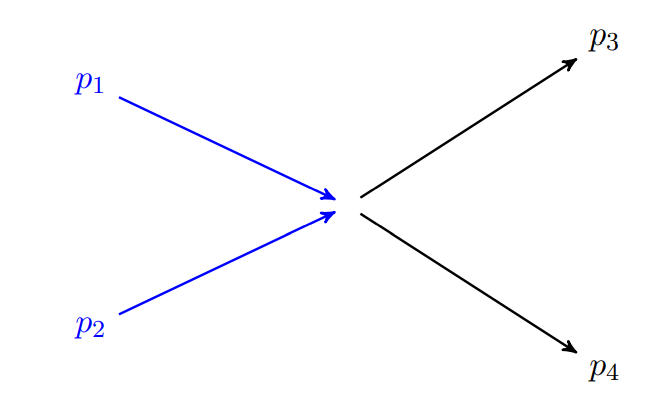Figure 3. The collision processes of classical particles and fermions

We will try to apply the above argument for a gas of fermions. For fermions, we cannot consider the collisions of each quantum particle as in the case of classical gas. We then modify the argument as follows: Suppose that two microscopic boxes with momenta p_1 and p_2 collide and after the collision some particles will move to the new boxes p_3 and p_4. As a result, we will have something similar to f(t,p_3)f(t,p_4)-f(t,p_1)f(t,p_2) in the collision operator. However, fermions follow the Pauli Exclusion Principle, which says that if the boxes p_3 and p_4 are already full, then particles cannot move from p_1 and p_2 to p_3 and p_4. We therefore modify the collision as f(t,p_3)f(t,p_4)(1-f(t,p_1))(1-f(t,p_2))-f(t,p_1)f(t,p_2)(1-f(t,p_3))(1-f(t,p_4)), where the factor 1-f(t,p_i) indicates the fullness of the box p_i. The quantum Boltzmann equation for fermions can be written as follows
\begin{aligned} &\ \frac{\text{d}f}{\text{d}t}(t,p_1) \\ = &\ \int_{\mathbb{R}^9}K(p_1,p_2,p_3,p_4)\delta(p_1+p_2-p_3-p_4)\delta(\mathcal{E}_{p_1} + \mathcal{E}_{p_2}- \mathcal{E}_{p_3} - \mathcal{E}_{p_4})\times \\ & \ \times[f(t,p_3)f(t,p_4)(1-f(t,p_1))(1-f(t,p_2))-f(t,p_1)f(t,p_2)(1-f(t,p_3))(1-f(t,p_4))] dp_2dp_3dp_4. \end{aligned}

The question now is how to adapt the above arguments to the case of C_{12} (see Figure 4). Suppose again that two microscopic boxes with momenta p_1 and p_4 collide but the box p_4 is hidden inside the condensate: we have a collision between p_1 and the condensate. After the collision some particles will move to the new boxes p_2 and p_3. Similar as above, in the collision operator, we will have something similar to f(t,p_2)f(t,p_3)-f(t,p_1). If we apply the same argument as above, the collision operator is proportional to f(t,p_2)f(t,p_3)(1-f(t,p_1))-f(t,p_1)(1-f(t,p_2))(1-f(t,p_3)). However, different from fermions, bosons do not follow the Pauli Exclusion Principle: the bosons in p_i are very friendly and they are trying to attract their friends to their home (Note that the fermions in p_i are selfish and do not welcome their friends to share their home). Therefore, instead of multiplying with 1-f(t,p_i), we use the factor 1+f(t,p_i) and modify the collision as f(t,p_2)f(t,p_3)(1+f(t,p_1))-f(t,p_1)(1+f(t,p_2))(1+f(t,p_3)). The conservation of momentum and energy in this case are p_1=p_2+p_3 and \mathcal{E}_{p_1}=\mathcal{E}_{p_2} +\mathcal{E}_{p_3}. The collision operator should contain
\begin{aligned} & \ \delta(p_1-p_2-p_3)\delta(\mathcal{E}_{p_1}-\mathcal{E}_{p_2}-\mathcal{E}_{p_3})\times\\\ &\ \times[f(t,p_2)f(t,p_3)(1+f(t,p_1))-f(t,p_1)(1+f(t,p_2))(1+f(t,p_3))] \end{aligned} (3)

Denoting this case as p_1\to p_2+p_3, we observe that there are still other two cases p_2\to p_1+p_3 and p_3\to p_1+p_2, that gives
\begin{aligned} & \ -\delta(p_2-p_1-p_3)\delta(\mathcal{E}_{p_2}-\mathcal{E}_{p_1}-\mathcal{E}_{p_3})\times\\\ &\ \times[f(t,p_1)f(t,p_3)(1+f(t,p_2))-f(t,p_2)(1+f(t,p_1))(1+f(t,p_3))] \end{aligned}

and

\begin{aligned} & \ \delta(p_3-p_2-p_1)\delta(\mathcal{E}_{p_3}-\mathcal{E}_{p_2}-\mathcal{E}_{p_1})\times\\\ &\ \times[f(t,p_3)f(t,p_2)(1+f(t,p_1))-f(t,p_3)(1+f(t,p_2))(1+f(t,p_1))]. \end{aligned}

Due to the symmetry of p_2 and p_3, we can combine them into
\begin{aligned} & \ -2\delta(p_2-p_1-p_3)\delta(\mathcal{E}_{p_2}-\mathcal{E}_{p_1}-\mathcal{E}_{p_3})\times\\\ &\ \times[f(t,p_1)f(t,p_3)(1+f(t,p_2))-f(t,p_2)(1+f(t,p_1))(1+f(t,p_3))] \end{aligned} (4)
Putting (3)) and (4) together, (1) then follows.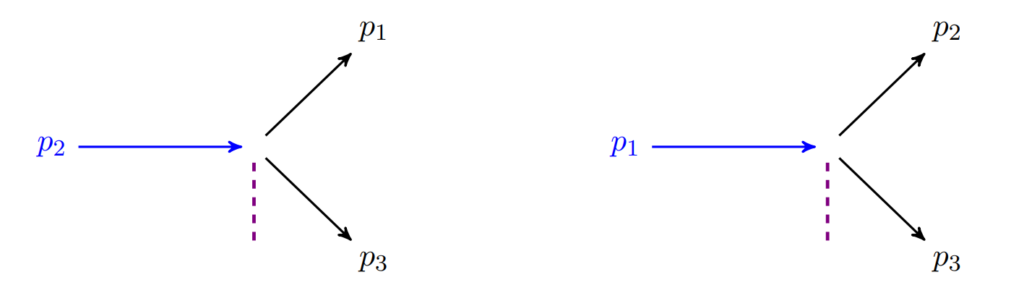Figure 4. The operator C_12

#### References

 R. Alonso, I. M. Gamba, and M.-B. Tran. The cauchy problem and bec stability for the quantum boltzmann-condensation system for bosons at very low temperature. arXiv preprint arXiv:1609.07467.
 M.H. Anderson, J.R. Ensher, M.R. Matthews, C.E. Wieman, and E.A. Cornell. Observation of Bose-Einstein Condensation in a dilute atomic vapor. Science, 269(5221):198–201, 1995.
 G. Craciun and M.-B. Tran. A reaction network approach to the convergence to equilibrium of quantum boltzmann equations for bose gases. ESAIM: Control, Optimisation and Calculus of Variations – Special Issue in honor of Enrique Zuazua, 2021.
 K. B. Davis, M.-O. Mewes, M. R. Andrews, N. J. van Druten, D. S. Durfee, D. M. Kurn, and W. Ketterle. Bose-Einstein condensation in a gas of sodium atoms. Physical review letters, 75(22):3969, 1995.
 A. Griffin, T. Nikuni, and E. Zaremba. Bose-condensed gases at finite temperatures. Cambridge University Press, Cambridge, 2009.
 S. Jin and M.-B. Tran. Quantum hydrodynamic approximations to the finite temperature trapped bose gases. Physica D: Nonlinear Phenomena, 380:45–57, 2018.
 T. R. Kirkpatrick and J. R. Dorfman. Transport coefficients in a dilute but condensed bose gas. J. Low Temp. Phys., 58:399–415, 1985.
 T. R. Kirkpatrick and J. R. Dorfman. Transport in a dilute but condensed nonideal bose gas: Kinetic equations. J. Low Temp. Phys., 58:301–331, 1985.
 Y. Pomeau and M.-B. Tran. Statistical Physics of Non Equilibrium Quantum Phenomena, volume 967 of Lecture Notes In Physics. Springer International Publishing, 2019.
 L. E. Reichl and M.-B. Tran. A kinetic equation for ultra-low temperature Bose–Einstein condensates. Journal of Physics A: Mathematical and Theoretical, 52(6):063001, 2019.
 A. Soffer and M.-B. Tran. On coupling kinetic and Schrödinger equations. Journal of Differential Equations, 265(5):2243–2279, 2018.
 A. Soffer and M.-B. Tran. On the dynamics of finite temperature trapped bose gases. Advances in Mathematics, 325:533–607, 2018.
 T. T. Nguyen and M.-B. Tran. Uniform in time lower bound for solutions to a quantum boltzmann equation of bosons. Archive for Rational Mechanics and Analysis, 231:63–89, 2019.
 M.-B. Tran and Y. Pomeau. Boltzmann-type collision operators for Bogoliubov excitations of Bose-Einstein condensates: A unified framework. Physical Review E 101 (3), 032119, 2020.
 M.-B. Tran and Y. Pomeau. On a thermal cloud – Bose-Einstein condensate coupling system. European Physical Journal Plus, 136, 502, 2021.
 E. Zaremba, T. Nikuni, and A. Griffin. Dynamics of trapped bose gases at finite temperatures. J. Low Temp. Phys., 116:277–345, 1999.

### You might like!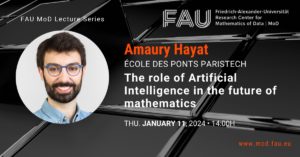#### FAU MoD Lecture: The role of Artificial Intelligence in the future of mathematics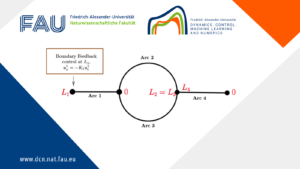#### Limits of the stabilization of a networked hyperbolic system with a circle